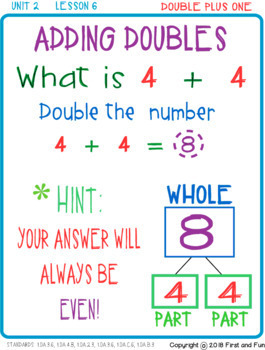# ADDING DOUBLES AND DOUBLE PLUS 1 WORKSHEETS EXIT TICKET AND POSTERS i READY COMMSubject
Resource Type
File Type
PDF (169 MB|28 pages)
Standards
\$3.50
Also included in:
1. You can save by downloading this bundle instead of the individual packs. They are bundled by unit and include all lessons contained in that unit.This is extra practice for i Ready Math First as well as any other First or Second Grade math curriculum. This product is NOT endorsed by Curriculum Associ
\$18.50
\$16.50
Save \$2.00
2. You can save by downloading this bundle instead of the individual packs. They are bundled by unit and include all lessons contained in that unit. This Bundle contains ALL Extra Practice Packs for the ENTIRE year! All 35 Lessons are included.This is extra practice for i Ready Math First Grade as wel
\$111.50
\$85.00
Save \$26.50
• Product Description
• Standards

ADDING DOUBLES AND DOUBLE PLUS 1 WORKSHEETS EXIT TICKET AND POSTERS i READY COMMON CORE

This pack is a great addition to any Math Curriculum for kinder and first grade. There are 8 Strategy Vocabulary Posters to post in the classroom. There are four posters for Adding Double and 4 posters for Adding Double+1. There are 19 differentiated worksheets total. Four of the worksheets are Exit Tickets with “I Can Statements”. Two of the Exit tickets have an answer key, so you can place at a Math Center.

This is extra practice for i Ready Math First Grade as well as any other First or Second Grade math curriculum.

This file is an independent product and is not affiliated with, nor has been authorized, sponsored, or otherwise approved by Pearson®, Always Learning®, Scott Foresman®, Pearson Scott Foresman®, or enVisionMath®, Curriculum Associates®

Unit 2: Learn Facts to 10, Lesson 6 Topic: Double and Double Plus One

ALSO AVAILABLE ARE UNIT 1 Lesson 1 Through Lesson 5 in MY STORE.

There will be more Units AND Lessons to come...

Remember this is intended for one classroom only. If you enjoy my product please consider following me on Teacher Pay Teachers store and rate my down load.
Please email me if you have any question. I can adjust any part of the product to meet the needs of your students.

**Remember to leave feedback to earn your TPT Credits!**

* THIS CONTENT IS NEITHER CREATED NOR ENDORSED BY i READY / CURRICULUM ASSOCIATES

Understand the meaning of the equal sign, and determine if equations involving addition and subtraction are true or false. For example, which of the following equations are true and which are false? 6 = 6, 7 = 8 - 1, 5 + 2 = 2 + 5, 4 + 1 = 5 + 2.
Add and subtract within 20, demonstrating fluency for addition and subtraction within 10. Use strategies such as counting on; making ten (e.g., 8 + 6 = 8 + 2 + 4 = 10 + 4 = 14); decomposing a number leading to a ten (e.g., 13 - 4 = 13 - 3 - 1 = 10 - 1 = 9); using the relationship between addition and subtraction (e.g., knowing that 8 + 4 = 12, one knows 12 - 8 = 4); and creating equivalent but easier or known sums (e.g., adding 6 + 7 by creating the known equivalent 6 + 6 + 1 = 12 + 1 = 13).
Apply properties of operations as strategies to add and subtract. If 8 + 3 = 11 is known, then 3 + 8 = 11 is also known. (Commutative property of addition.) To add 2 + 6 + 4, the second two numbers can be added to make a ten, so 2 + 6 + 4 = 2 + 10 = 12. (Associative property of addition.)
Solve word problems that call for addition of three whole numbers whose sum is less than or equal to 20, e.g., by using objects, drawings, and equations with a symbol for the unknown number to represent the problem.
Use addition and subtraction within 20 to solve word problems involving situations of adding to, taking from, putting together, taking apart, and comparing, with unknowns in all positions, e.g., by using objects, drawings, and equations with a symbol for the unknown number to represent the problem.
Total Pages
28 pages